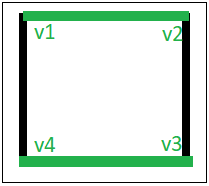# Edge Coloring of a Graph

In graph theory, edge coloring of a graph is an assignment of “colors” to the edges of the graph so that no two adjacent edges have the same color with an optimal number of colors. Two edges are said to be adjacent if they are connected to the same vertex. There is no known polynomial time algorithm for edge-coloring every graph with an optimal number of colors. Nevertheless, a number of algorithms have been developed that relax one or more of these criteria, they only work on a subset of graphs, or they do not always use an optimal number of colors, or they do not always run in polynomial time.

Examples:

```Input : u1 = 1, v1 = 4
u2 = 1, v2 = 2
u3 = 2, v3 = 3
u4 = 3, v4 = 4
Output : Edge 1 is of color 1
Edge 2 is of color 2
Edge 3 is of color 1
Edge 4 is of color 2

The above input shows the pair of vertices(ui, vi)
who have an edge between them. The output shows the color
assigned to the respective edges.
```

## Recommended: Please try your approach on {IDE} first, before moving on to the solution.Edge colorings are one of several different types of graph coloring problems. The above figure of a Graph shows an edge coloring of a graph by the colors green and black, in which no adjacent edge have the same color.

Below is an algorithm to solve the edge coloring problem which may not use an optimal number of colors:
Algorithm:

1. Use BFS traversal to start traversing the graph.
2. Pick any vertex and give different colors to all of the edges connected to it, and mark those edges as colored.
3. Traverse one of it’s edges.
4. Repeat step to with a new vertexd until all edges are colored.

Below is the implementaion of above approach:

 `// C++ program to illustrate Edge Coloring ` `#include ` `using` `namespace` `std; ` ` `  `// function to determine the edge colors ` `void` `colorEdges(``int` `ptr, vector > >& gra, ` `                ``vector<``int``>& edgeColors, ``bool` `isVisited[]) ` `{ ` `    ``queue<``int``> q; ` `    ``int` `c = 0; ` ` `  `    ``set<``int``> colored; ` ` `  `    ``// return if isVisited[ptr] is true ` `    ``if` `(isVisited[ptr]) ` `        ``return``; ` ` `  `    ``// Mark the current node visited ` `    ``isVisited[ptr] = 1; ` ` `  `    ``// Traverse all edges of current vertex ` `    ``for` `(``int` `i = 0; i < gra[ptr].size(); i++) { ` `        ``// if already colored, insert it into the set ` `        ``if` `(edgeColors[gra[ptr][i].second] != -1) ` `            ``colored.insert(edgeColors[gra[ptr][i].second]); ` `    ``} ` ` `  `    ``for` `(``int` `i = 0; i < gra[ptr].size(); i++) { ` `        ``// if not visited, inset into the queue ` `        ``if` `(!isVisited[gra[ptr][i].first]) ` `            ``q.push(gra[ptr][i].first); ` ` `  `        ``if` `(edgeColors[gra[ptr][i].second] == -1) { ` `            ``// if col vector -> negative ` `            ``while` `(colored.find(c) != colored.end()) ` ` `  `                ``// increment the color ` `                ``c++; ` ` `  `            ``// copy it in the vector ` `            ``edgeColors[gra[ptr][i].second] = c; ` ` `  `            ``// then add it to the set ` `            ``colored.insert(c); ` `            ``c++; ` `        ``} ` `    ``} ` ` `  `    ``// while queue's not empty ` `    ``while` `(!q.empty()) { ` `        ``int` `temp = q.front(); ` `        ``q.pop(); ` ` `  `        ``colorEdges(temp, gra, edgeColors, isVisited); ` `    ``} ` ` `  `    ``return``; ` `} ` ` `  `// Driver Function ` `int` `main() ` `{ ` `    ``set<``int``> empty; ` ` `  `    ``// declaring vector of vector of pairs, to define Graph ` `    ``vector > > gra; ` ` `  `    ``vector<``int``> edgeColors; ` ` `  `    ``bool` `isVisited = { 0 }; ` ` `  `    ``// Enter the Number of Vertices ` `    ``// and the number of edges ` `    ``int` `ver = 4; ` `    ``int` `edge = 4; ` ` `  `    ``gra.resize(ver); ` `    ``edgeColors.resize(edge, -1); ` ` `  `    ``// Enter edge & vertices of edge ` `    ``// x--; y--; ` `    ``// Since graph is undirected, push both pairs ` `    ``// (x, y) and (y, x) ` `    ``// graph[x].push_back(make_pair(y, i)); ` `    ``// graph[y].push_back(make_pair(x, i)); ` `    ``gra.push_back(make_pair(1, 0)); ` `    ``gra.push_back(make_pair(0, 0)); ` ` `  `    ``gra.push_back(make_pair(2, 1)); ` `    ``gra.push_back(make_pair(1, 1)); ` ` `  `    ``gra.push_back(make_pair(3, 2)); ` `    ``gra.push_back(make_pair(2, 2)); ` ` `  `    ``gra.push_back(make_pair(3, 3)); ` `    ``gra.push_back(make_pair(0, 3)); ` ` `  `    ``colorEdges(0, gra, edgeColors, isVisited); ` ` `  `    ``// printing all the edge colors ` `    ``for` `(``int` `i = 0; i < edge; i++) ` `        ``cout << ``"Edge "` `<< i + 1 << ``" is of color "` `             ``<< edgeColors[i] + 1 << ``"\n"``; ` ` `  `    ``return` `0; ` `} `

Output:

```Edge 1 is of color 1
Edge 2 is of color 2
Edge 3 is of color 1
Edge 4 is of color 2
```

Reference : https://en.wikipedia.org/wiki/Edge_coloring

Don’t stop now and take your learning to the next level. Learn all the important concepts of Data Structures and Algorithms with the help of the most trusted course: DSA Self Paced. Become industry ready at a student-friendly price.

My Personal Notes arrow_drop_upCheck out this Author's contributed articles.

If you like GeeksforGeeks and would like to contribute, you can also write an article using contribute.geeksforgeeks.org or mail your article to contribute@geeksforgeeks.org. See your article appearing on the GeeksforGeeks main page and help other Geeks.

Please Improve this article if you find anything incorrect by clicking on the "Improve Article" button below.

Article Tags :
Practice Tags :

3

Please write to us at contribute@geeksforgeeks.org to report any issue with the above content.